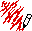EECS 311: Space Complexity Home Course Info Links Grades Lectures Newsgroup Homework Exams

The book doesn't really talk much about space complexity. Google "algorithm space complexity" and you'll see many online sites only paying lip service to the concept. The few sites that do talk about space complexity are very formal, describing things in terms of Turing machines, which is beyond the scope of this course. So this is the Bluffer's Guide to Space Complexity.

Space complexity is a measure of the amount of working storage an algorithm needs. That means how much memory, in the worst case, is needed at any point in the algorithm. As with time complexity, we're mostly concerned with how the space needs grow, in big-Oh terms, as the size N of the input problem grows.

```int sum(int x, int y, int z) {
int r = x + y + z;
return r;
}```

requires 3 units of space for the parameters and 1 for the local variable, and this never changes, so this is O(1).

```int sum(int a[], int n) {
int r = 0;
for (int i = 0; i < n; ++i) {
r += a[i];
}
return r;
}```

requires N units for a, plus space for n, r and i, so it's O(N). What are the space complexities of these next two functions?

```void matrixAdd(int a[], int b[], int c[], int n) {
for (int i = 0; i < n; ++i) {
c[i] = a[i] + b[j]
}
}```
```void matrixMultiply(int a[], int b[], int c[][], int n) { // not legal C++
for (int i = 0; i < n; ++i) {
for (int j = 0; j < n; ++j) {
c[i] = a[i] + b[j];
}
}
}```

If a function A uses M units of its own space (local variables and parameters), and it calls a function B that needs N units of local space, then A overall needs M + N units of temporary workspace. What if A calls B 3 times? When a function finishes, its space can be reused, so if A calls B 3 times, it still only needs M + N units of workspace.

What if A calls itself recursively N times? Then its space can't be reused because every call is still in progress, so it needs O(N2) units of workspace.

But be careful here. If things are passed by pointer or reference, then space is shared. If A passes a C-style array to B, there is no new space allocated. If A passes a C++ object by reference to B, there is no new space. What if A passes a vector or string by value? Most likely, new space will be allocated. Some C++ compilers try to avoid this for strings, using a technique called copy-on-write, but this is no longer a common thing to do.

Comments?Send mail to c-riesbeck@northwestern.edu.